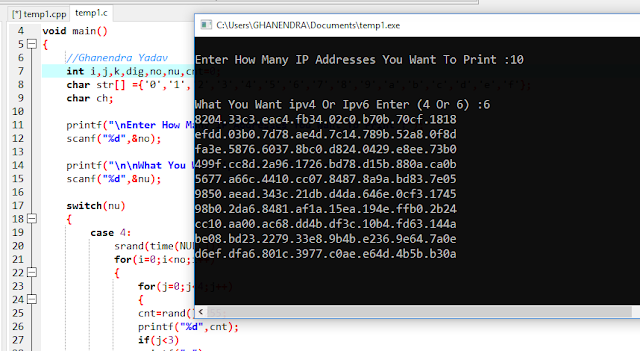# C Program To Generate IP Addresses(IPv4 & IPv6) Using For Loop

Write a C Program To Generate IP Addresses (Internet Protocol IPv4 & IPv6 ) Using For Loop. Generate IP Address in C. Logic is very simple first print a random Number so I use the rand() Function But always shows the same value if you run your code again and again it shows the same or repeat Output that's why I use stand(). So to print IPv4 we need to print multiple of 4 random numbers cause we need output in this format.

IPv4: What is IPv4 Format?

64.92.8.122 This is an IPv4 Format or Number from 0 to 255 or you can say 0.0.0.0 to 255.255.255.255 Below Code is Printing an IPv4.

``````srand(time(NULL));
for (i = 0; i < no; i++) {
for (j = 0; j < 4; j++) {
cnt = rand() % 255;
printf("%d", cnt);
if (j < 3)
printf(".");
}
printf("\n");
}``````

IPv6: What is IPv6 Format?

5677.a66c.4410.cc07.8487.8a9a.bd83.7e05 This is an IPv6 Format or we also use hexadecimal Numbers IPv6 that's a reason IPv6 is More Secure Than IPv4. Below Code For IPv6 But we also Use A Character array See the Full Code.

``````srand(time(NULL));
for (i = 0; i < no; i++) {
for (j = 0; j < 8; j++) {
for (k = 0; k < 4; k++) {
ch = str[rand() % 16];
printf("%c", ch);
}
if (j < 7)
printf(".");
}
printf("\n");
}``````

Read: C Program To Print A Calendar By Taking Input From User Using Loop

## C Program To Generate IP Addresses

``````#include<stdio.h>

#include<string.h>

#include<math.h>

void main() {
/*C Program To Generate IP Addresses*/
int i, j, k, dig, no, nu, cnt = 0;
char str[] = {
'0',
'1',
'2',
'3',
'4',
'5',
'6',
'7',
'8',
'9',
'a',
'b',
'c',
'd',
'e',
'f'
};
char ch;

printf("\nEnter How Many IP Addresses You Want To Print: ");
scanf("%d", & no);

printf("\n\nEnter 4 for IPv4 and 6 for IPv6: \n");
scanf("%d", & nu);

switch (nu) {
case 4:
srand(time(NULL));
for (i = 0; i < no; i++) {
for (j = 0; j < 4; j++) {
cnt = rand() % 255;
printf("%d", cnt);
if (j < 3)
printf(".");
}
printf("\n");
}
break;
case 6:
srand(time(NULL));
for (i = 0; i < no; i++) {
for (j = 0; j < 8; j++) {
for (k = 0; k < 4; k++) {
ch = str[rand() % 16];
printf("%c", ch);
}
if (j < 7)
printf(".");
}
printf("\n");
}
break;
default:
printf("\nEnter IPv Either 4 or 6\n\n");
}
}``````